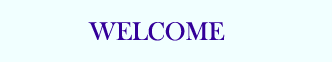TUTORIALS

## PHP Basic

PHP HOME
PHP Intro
PHP Install
PHP Syntax
PHP Variables
PHP String
PHP Operators
PHP If...Else
PHP Switch
PHP Arrays
PHP While Loops
PHP For Loops
PHP Functions
PHP Forms
PHP \$_GET
PHP \$_POST

PHP Date
PHP Include
PHP File
PHP Sessions
PHP E-mail
PHP Secure E-mail
PHP Error
PHP Exception
PHP Filter

## PHP Database

MySQL Introduction
MySQL Connect
MySQL Create
MySQL Insert
MySQL Select
MySQL Where
MySQL Order By
MySQL Update
MySQL Delete
PHP ODBC

XML Expat Parser
XML DOM
XML SimpleXML

AJAX Intro
AJAX PHP
AJAX Database
AJAX XML
AJAX Live Search
AJAX Poll

PHP Array
PHP Calendar
PHP Date
PHP Directory
PHP Error
PHP Filesystem
PHP Filter
PHP FTP
PHP HTTP
PHP Libxml
PHP Mail
PHP Math
PHP Misc
PHP MySQL
PHP SimpleXML
PHP String
PHP XML
PHP Zip

PHP Quiz
PHP Exam

# PHP Arrays

An array stores multiple values in one single variable.

## What is an Array?

A variable is a storage area holding a number or text. The problem is, a variable will hold only one value.

An array is a special variable, which can store multiple values in one single variable.

If you have a list of items (a list of car names, for example), storing the cars in single variables could look like this:

 \$cars1="Saab"; \$cars2="Volvo"; \$cars3="BMW";

However, what if you want to loop through the cars and find a specific one? And what if you had not 3 cars, but 300?

The best solution here is to use an array!

An array can hold all your variable values under a single name. And you can access the values by referring to the array name.

Each element in the array has its own index so that it can be easily accessed.

In PHP, there are three kind of arrays:

• Numeric array - An array with a numeric index
• Associative array - An array where each ID key is associated with a value
• Multidimensional array - An array containing one or more arrays

## Numeric Arrays

A numeric array stores each array element with a numeric index.

There are two methods to create a numeric array.

1. In the following example the index are automatically assigned (the index starts at 0):

 \$cars=array("Saab","Volvo","BMW","Toyota");

2. In the following example we assign the index manually:

 \$cars="Saab"; \$cars="Volvo"; \$cars="BMW"; \$cars="Toyota";

### Example

In the following example you access the variable values by referring to the array name and index:



The code above will output:

 Saab and Volvo are Swedish cars.

## Associative Arrays

An associative array, each ID key is associated with a value.

When storing data about specific named values, a numerical array is not always the best way to do it.

With associative arrays we can use the values as keys and assign values to them.

### Example 1

In this example we use an array to assign ages to the different persons:

 \$ages = array("Peter"=>32, "Quagmire"=>30, "Joe"=>34);

### Example 2

This example is the same as example 1, but shows a different way of creating the array:

 \$ages['Peter'] = "32"; \$ages['Quagmire'] = "30"; \$ages['Joe'] = "34";

The ID keys can be used in a script:



The code above will output:

 Peter is 32 years old.

## Multidimensional Arrays

In a multidimensional array, each element in the main array can also be an array. And each element in the sub-array can be an array, and so on.

### Example

In this example we create a multidimensional array, with automatically assigned ID keys:

 \$families = array   (   "Griffin"=>array   (   "Peter",   "Lois",   "Megan"   ),   "Quagmire"=>array   (   "Glenn"   ),   "Brown"=>array   (   "Cleveland",   "Loretta",   "Junior"   )   );

The array above would look like this if written to the output:

 Array ( [Griffin] => Array   (    => Peter    => Lois    => Megan   ) [Quagmire] => Array   (    => Glenn   ) [Brown] => Array   (    => Cleveland    => Loretta    => Junior   ) )

### Example 2

Lets try displaying a single value from the array above:

 echo "Is " . \$families['Griffin'] . " a part of the Griffin family?";

The code above will output:

 Is Megan a part of the Griffin family?

## Complete PHP Array Reference

For a complete reference of all array functions, go to our complete PHP Array Reference.

The reference contains a brief description, and examples of use, for each function!